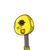# one upon two multiplied by five upon six-plus one upon three multiplied by one upon four

one upon two multiplied by five upon six-plus one upon three multiplied by one upon four

### 1 thought on “one upon two multiplied by five upon six-plus one upon three multiplied by one upon four<br /><br />”

1.½×⅚+⅓×¼ = 5/12 + 1/12 = 6/12=½